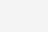# C语言的整型溢出问题

#### 什么是整型溢出

C语言的整型问题相信大家并不陌生了。对于整型溢出，分为无符号整型溢出和有符号整型溢出。

```unsigned char x = 0xff;
printf("%d\n", ++x);```

```signed char x =0x7f; //注：0xff就是-1了，因为最高位是1也就是负数了
printf("%d\n", ++x);```

```signed char x = 0x7f;
signed char y = 0x05;
signed char r = x * y;
printf("%d\n", r);```

#### 整型溢出的危害

##### 示例一：整形溢出导致死循环
```... ...
... ...
short len = 0;
... ...
while(len< MAX_LEN) {
buf += len;
}```
##### 示例二：整形转型时的溢出
```int copy_something(char *buf, int len)
{
#define MAX_LEN 256
char mybuf[MAX_LEN];
... ...
... ...

if(len > MAX_LEN){ // <---- 
return -1;
}

return memcpy(mybuf, buf, len);
}```
##### 示例三：分配内存

```nresp = packet_get_int();
if (nresp > 0) {
response = xmalloc(nresp*sizeof(char*));
for (i = 0; i < nresp; i++)
response[i] = packet_get_string(NULL);
}```

##### 示例四：缓冲区溢出导致安全问题
```int func(char *buf1, unsigned int len1,
char *buf2, unsigned int len2 )
{
char mybuf;

if((len1 + len2) > 256){    //<--- 
return -1;
}

memcpy(mybuf, buf1, len1);
memcpy(mybuf + len1, buf2, len2);

do_some_stuff(mybuf);

return 0;
}```
##### 示例五：size_t 的溢出
```for (int i= strlen(s)-1;  i>=0; i--)  { ... }
for (int i=v.size()-1; i>=0; i--)  { ... }```

#### 关于编译器的行为

##### 编译器优化

```int len;
char* data;

if (data + len < data){
printf("invalid len\n");
exit(-1);
}```

```7           int len = 10;
8           char* data = (char *)malloc(len);
0x00000000004004d4 <+4>:     mov    \$0xa,%edi
0x00000000004004d9 <+9>:     callq  0x4003b8 <malloc@plt>

9
10          if (data + len < data){
11              printf("invalid len\n");
12              exit(-1);
13          }
14
15      }
0x00000000004004e2 <+18>:    retq```

```if ((uintptr_t)data + len < (uintptr_t)data){
... ...
}```##### 花絮：编译器的彩蛋

```execl("/usr/games/hack", "#pragma", 0); // try to run the game NetHack
execl("/usr/games/rogue", "#pragma", 0); // try to run the game Rogue
// try to run the Tower's of Hanoi simulation in Emacs.
execl("/usr/new/emacs", "-f","hanoi","9","-kill",0);
execl("/usr/local/emacs","-f","hanoi","9","-kill",0); // same as above
fatal("You are in a maze of twisty compiler features, all different");```

```void foo(int m, int n)
{
size_t s = m + n;
.......
}```

```void foo(int m, int n)
{
size_t s = m + n;
if ( m>0 && n>0 && (SIZE_MAX - m < n) ){
//error handling...
}
}```

1）检查的太晚了，if之前编译器的undefined行为就已经出来了（你不知道什么会发生）。

2）就像前面说的一样，(SIZE_MAX – m < n) 可能会被编译器优化掉。

3）另外，SIZE_MAX是size_t的最大值，size_t在64位系统下是64位的，严谨点应该用INT_MAX或是UINT_MAX

所以，正确的代码应该是下面这样：

```void foo(int m, int n)
{
size_t s = 0;
if ( m>0 && n>0 && ( UINT_MAX - m < n ) ){
//error handling...
return;
}
s = (size_t)m + (size_t)n;
}```##### 二分取中搜索算法中的溢出

```int binary_search(int a[], int len, int key)
{
int low = 0;
int high = len - 1;

while ( low<=high ) {
int mid = (low + high)/2;
if (a[mid] == key) {
return mid;
}
if (key < a[mid]) {
high = mid - 1;
}else{
low = mid + 1;
}
}
return -1;
}```

1） 我们应该用size_t来做len, low, high, mid这些变量的类型。没错，应该是这样的。但是如果这样，你要小心第四行 int high = len -1; 如果len为0，那么就“high大发了”。

2） 无论你用不用size_t。我们在计算mid = (low+high)/2; 的时候，(low + high) 都可以溢出。正确的写法应该是：

`int mid = low + (high - low)/2;`

```#include <limits.h>

void f(signed int si_a, signed int si_b) {
signed int sum;
if (((si_b > 0) && (si_a > (INT_MAX - si_b))) ||
((si_b < 0) && (si_a < (INT_MIN - si_b)))) {
/* Handle error */
return;
}
sum = si_a + si_b;
}```

```void func(signed int si_a, signed int si_b)
{
signed int result;
if (si_a > 0) {  /* si_a is positive */
if (si_b > 0) {  /* si_a and si_b are positive */
if (si_a > (INT_MAX / si_b)) {
/* Handle error */
}
} else { /* si_a positive, si_b nonpositive */
if (si_b < (INT_MIN / si_a)) {
/* Handle error */
}
} /* si_a positive, si_b nonpositive */
} else { /* si_a is nonpositive */
if (si_b > 0) { /* si_a is nonpositive, si_b is positive */
if (si_a < (INT_MIN / si_b)) {
/* Handle error */
}
} else { /* si_a and si_b are nonpositive */
if ( (si_a != 0) && (si_b < (INT_MAX / si_a))) {
/* Handle error */
}
} /* End if si_a and si_b are nonpositive */
} /* End if si_a is nonpositive */

result = si_a * si_b;
}```

(0)

### 相关推荐

• #### sed的模式匹配用法探讨

原创作品，允许转载，转载时请务必以超链接形式标明文章 原始出处 、作者信息和本声明。否则将追究法律责任。http://nolinux.blog.51cto.com/4824967/1405753 [root@sunsky Desktop]# cat sunsky a b c d e f [root@sunsk…

Linux干货 2016-08-15
• #### Dell R720 安装debian系统编译网卡驱动

最近比较忙，视频也没怎么看，下面是之前工作中遇到的问题，事后做的记录，中间解决过程参考了网上的相关资料，现在一并贴出来。 需求：Dell R720 安装debian系统 环境 硬件：Dell R720  软件：系统有debian 7.0.0和debian 6.0.10 1. debian 7.0.0 : 集成了DELL PCRE H710 Mini…

Linux干货 2015-08-04
• #### Linux基础——文件管理

Linux基础——文件管理 文件管理及常用命令详解       Linux文件系统是以/为基本的倒置树状结构，我们来进一步学习下文件或者目录的操作和管理命令：  目录相关操作：cd、pwd、mkdir、rmdir 目录及文件检索：ls 复制、移动、删除：cp、mv、rm…

Linux干货 2016-09-27
• #### rpm包管理与yum简要用法

linux程序包管理 程序包：源代码 –> 目标二进制格式（二进制程序、库文件、配置文件、帮助文件）–> 组织成一个或有限的几个包文件 程序包管理器：        debian –> dpkg   以“.deb”结尾 &nb…

Linux干货 2016-08-22
• #### mkdir用法实践

mkdir：make directories mkdir [OPTION]… DIRECTORY… -p: 自动按需创建父目录； -v: verbose，显示详细过程； -m MODE：直接给定权限；   1、创建/tmp目录下的：a_c,a_d, b_c, b_d ~]# mkdir -v /tmp/{a,b}_{c,d}…

Linux干货 2016-11-06
• #### 马哥教育网络班21期+第4周作业

1、复制/etc/skel目录为/home/tuser1,要求/home/tuser1及其内部文件的属组和其它用户均没有任何访问权限。 [root@localhost ~]# cp -r /etc/skel /home/ && mv /home/skel&nbsp…

Linux干货 2016-08-02